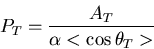## Outline of E246 experiments

In contrast to the previous in-flight-decay experiment, E246 employs the stopped K+ method. This technique enables a measurement with coverage of all directions in the decay phase space including kinematic regions with opposite PT. Thus, a double ratio measurement can be carried out with the consequence of very small systematic errors. A positive kaon beam with 660 MeV/c is slowed down and stopped in a target made of 256 scintillating fibers situated in the center of a superconducting toroidal spectrometer, which has a large solid angle and wide momentum range with 12 gaps and analyzes the μ+ momentum. A TOF system separates μ+ from e+. The π0 is detected as two photons or one photon with relatively large energy by a CsI(Tl) calorimeter consisting of 768 crystals. A K+μ3 decay is identified as coincident μ+ and π0 which satisfy certain kinematical conditions. The experimental setup is shown in setup pages.
The momentum analyzed μ+ stops in the polarimeter and the muon polarization is measured by means of decay positron asymmetry. If we look at events with pions in the forward(fwd) or backward(bwd) directions relative to the beam axis, the direction of PT is either in the clockwise (cw) or counter-clockwise (ccw) direction in the muon stoppers. PT is then measured as the asymmetry in cw and ccw positron emission. At the Al stopper a magnetic field is applied parallel to azimuthal PT to preserve it and also to rotate the radial in-plane polarization components. The positron asymmetry is then extracted using the following double ratio,It is noted here that several systematics such as the kaon stopping distribution in the target are cancelled out by taking the summation over the 12 gaps. Most of the remaining systematic errors can be further cancelled out in taking the second ratio. The fwd and bwd regions of π0 (for two photon events) and γ (for one photon events) were 0° < θπ0, γ < 70° and 110° < θπ0 < 180°, respectively, for the π0(or γ) polar angle from the figure of merit optimization. PT is then calculated asby using the analyzing power, α, and the kinematical attenuation factor, <cos θT>. Imξ can be further extracted from PT by dividing it by a sensitivity coefficient, Φ, determined in a Monte Carlo calculation as Imξ = PT.